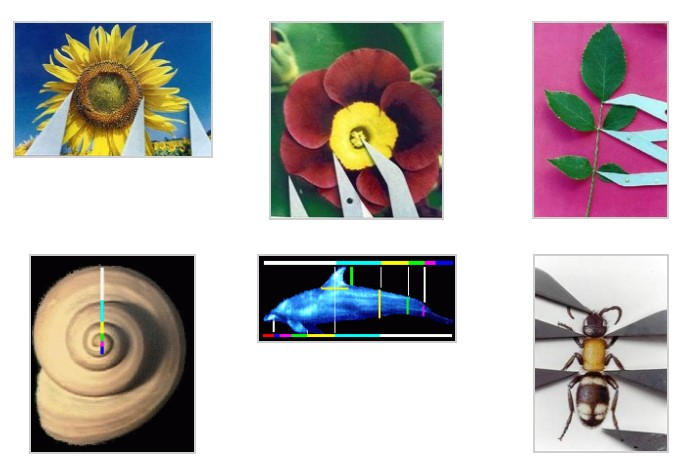## Phi the Key to Beauty

Phi appears in nature and the human body, as illustrated by the photos below.  Click on any image to zoom to full size.## Looking For Beauty

The Greeks said that all beauty is mathematics. If that is true then perhaps there is a mathematical code, formula, relationship or even a number that can describe facial beauty.

Historically many different numbers have been tried in an attempt to describe beauty however, only one mathematical relationship has been consistently and repeatedly reported to be present in beautiful things.

## The Golden Ratio

This particular relationship is the “Golden Ratio“. It is a mathematical ratio that seems to appear recurrently in beautiful things in nature as well as in other things that are seen as “Beautiful”.

The “Golden Ratio” is a mathematical ratio of 1.618:1, and the number 1.618 is called “Phi“.

Many things found in nature have parts that are in a ratio of 1.618:1 as seen above.

Images above demonstrating the golden ratio graphically with the multi-colored Golden Ruler are by Gary B Meisner of www.goldennumber.net.

Images above showing the use of the golden mean gauge (insects, plants, hand and teeth) are by Dr. Eddy Levin of www.goldenmeangauge.co.uk.

### Other Names For The Golden Ratio:

The ratio of 1:1.618 (The “Golden Ratio”) is also referred to by other names.
Some of these are:

The Phi Ratio

The Fibonacci Ratio

The Divine Ratio

The Golden Mean

The Golden Section

## The Golden Proportion

Two equal ratios are called a proportion. Since all golden ratios are equal to each other, two golden ratios shown as equaling each other are referred to as a “Golden Proportion”.

The term “Golden Proportion” is also referred to by other names including:

The Phi Proportion

The Fibonacci Proportion

The Divine Proportion